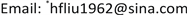1陆军工程大学基础部，江苏 南京

2电子科技大学计算机科学与工程学院，四川 成都1. 引言

2. 小区开放对路网通行能力指标影响度评估分析

2.1. 小区内部支路状况对城市路网影响的评价指标

1) 小区内部道路面积比率(URAR, Urban Road Area Ratio )

URAR = ∑ i = 1 n s i / s = ∑ i = 1 n l i d i / s (1)

2) 小区内部平均路网密度ρ

ρ = ∑ i = 1 n l i / s (2)

Relationship between road area ratio and branch scale of residential are

Relationship between average road network density and plot siz

3) 低质量支路所占比例q

q = ∑ j = 1 m b j / ∑ i = 1 n l i ,   m ≤ n (3)

4) 小区实际接收车流量l

l = v × ∏ i = 1 3 η i (4)

2.2. 小区周边路网状况对城市路网影响评估指标

5) 交叉路口饱和度S：是指交叉口实际交通量与通行能力之比：

S = V / C (5)

6) 路段相对延误率drR

a) 路段行驶时间 t L 。车辆行驶过路长为L的路段所需时间。这个量可以由现场实测，也可以通过关系值计算得出。该指标直接体现出行时耗和拥挤程度，同时也间接体现了小区附近的基础设施建设结构。

b) 路段平均速度 v L 。车辆通过路段时路段长度L与路段行驶时间 t L 之比，反映了交通流在特定路段空间上的运行状况，体现道路的通畅程度，计算公式如下：

v L = 60 × L / t L (6)

c) 出行速率u。车辆在特定路段上行驶时每公里所需要的时间平均值：

u = t L / L (7)

d) 延误值 d L 。实际出行时间 t L 与可接受出行时间 t L a 的差值。

d L = t L − t L a (8)

e) 总延误值 D L t 。在一定时间t内通过该路段的所有车辆延误之和：

D L t = ∑ i = 1 n d L i (9)

f) 绝对延误率 d r L 。即实际出行速率与可接受出行速率之差即路段时间损失率，其值可以用来评价交通改善方案的运行情况。

d r L = u − u a = ( t L − t L a ) / L = d L / L (10)

g) 路段相对延误率 d r R 。是一个无量纲的指标，用于比较不同路况之间拥堵情况的差异性。

d r R = ( t L − t L a ) / t L a = d L / t L a (11)

3. 开放小区路网评估指标的性能分析3.1. 基于主成分分析的路网指标性能评估

R = [ r 11 r 12 ⋯ r 16 r 21 r 22 ⋯ r 26 ⋮ ⋮ ⋱ ⋮ r 61 r 62 ⋯ r 66 ] (12)

r i j = ∑ k = 1 6 ( x k i − x i ¯ ) ( x k j − x j ¯ ) ∑ k = 1 6 ( x k i − x i ¯ ) 2 ∑ k = 1 6 ( x k j − x j ¯ ) 2 (13)

λ 1 ≥ λ 2 ≥ λ i ≥ λ 6 ≥ 0 (14)

Correlation coefficient matrix among 6 indexe
URARρqlSd r R
URAR10.90−0.450.76−0.56−0.72
ρ0.901−0.070.24−0.65−0.73
q−0.45−0.0710.210.540.66
l0.760.240.211−0.21−0.31
S−0.56−0.650.54−0.2110.78
d r R−0.72−0.730.66−0.310.781

6 contribution rate statistics of assessment factor

3.2. 基于相关性分析的路网指标性能评估

M I = ( P × v ¯ ) / ∑ i = 1 n γ Q i υ i (17)

Data statistics of indicator correlation matri
MIURARρqlSd r R
MI10.830.89−0.420.71−0.52−0.74
URAR0.8310.910.03−0.13−0.49−0.66
ρ0.890.911−0.060.25−0.63−0.74
q−0.420.03−0.0610.220.520.63
l0.71−0.130.250.221−0.22−0.30
S−0.52−0.49−0.630.52−0.2210.76
d r R−0.74−0.66−0.740.63−0.310.761

4. 小区开放对优化周边路网性能的建议

5. 结束语